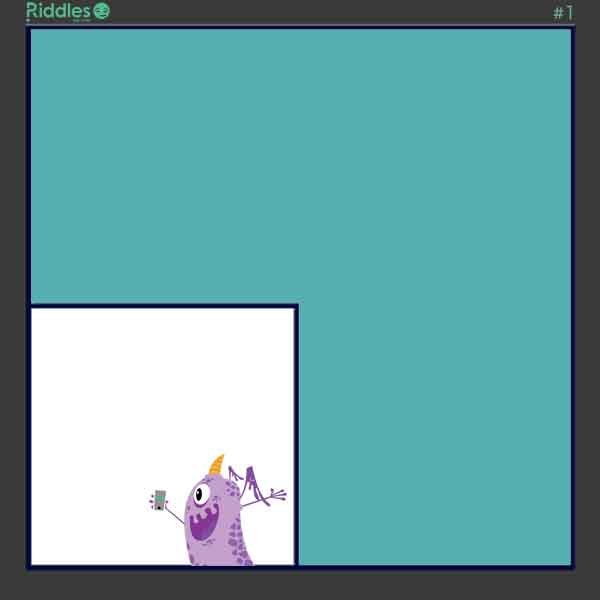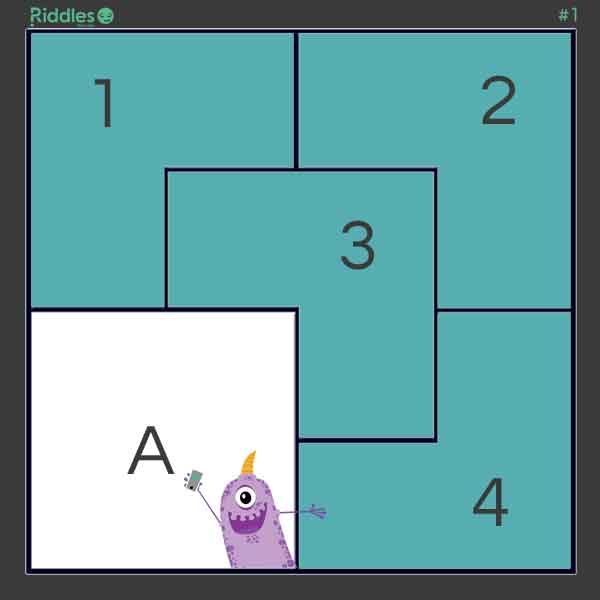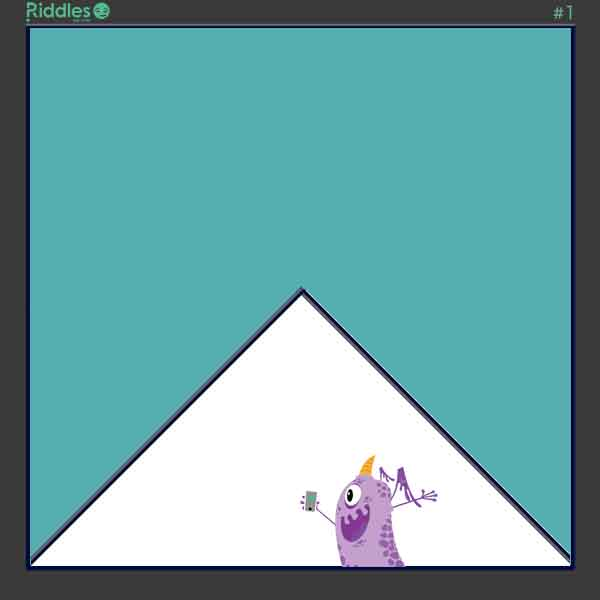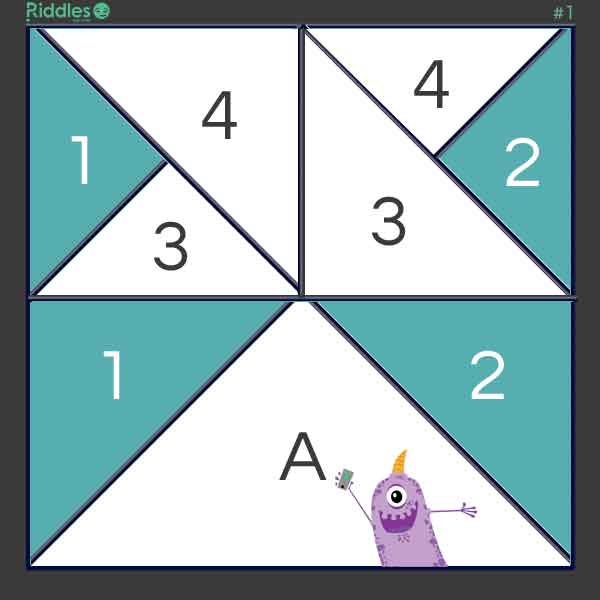# Carl Is Trying To Find Solutions To A Geometric Puzzle. He Has A Square Plot Of Land...

Author: Puzzle Games
3 years ago

Riddle: Carl is trying to find solutions to a geometric puzzle. He has a square plot of land that he needs to reserve 1/4 for himself and divide the remaining 3/4 equally and in a similar shape, among his 4 children. There are two possible solutions. Can you solve the puzzle?
Answer: Solution #1 - Squares First, Carl divides his as to reserve to himself one-fourth in the form of a square.Then, Carl takes the remaining 3/4 shape and scales it down by 1/4.  He then, multiplies the shape into 4 identically shaped pieces, and aranges them so that they fit into the original 3/4 shape.Solution #2 - Rectangles First, create a triangle that is 1/4 the size of the square.Now, with straight lines, create two squares. Proceed to disect the two squares with horizontal lines creating 4 triangles. Then, disect one of the resultuing triangles from each square.  The shape of land for each of his four children is divided evenly and is the same shape.VOTE
Source: https://www.riddles.com/1